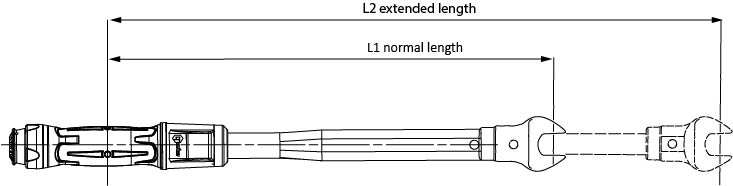# Torque Wrench Extension Formula

Norbar’s torque wrench extension calculator is now available as an app to download free on iPhone and Android. An invaluable tool for engineers, the torque extension app calculates the exact torque wrench setting value for required level of torque to be applied.

When you put an extension on a torque wrench (e.g. a crow foot or a dog bone) the torque applied to the fastener increases as the lever arm extends. The calculator will work out what value you should set into the wrench to achieve the required fastener torque.

The following formula has been used:

• M1 = M2 x L1 / L2

Where:

• M1 is the torque setting of the wrench
• M2 is the actual torque applied to the nut
• L1 is the normal length of the wrench
• L2 is the extended length of the wrench